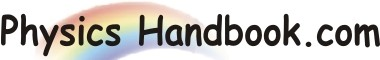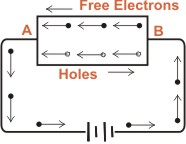HOME TOPICS DEFINITIONS TABLES LAWS INVENTIONS EXPERIMENTS QUIZ VIDEOS
 A B C D E F G H I J K L M N O P Q R S T U V W X Y Z
Instrinsic Semiconductor
A semiconductor in an extremely pure form is known as an instrinsic semiconductor.
In an instrinsic semiconductor, even at room temperature, hole-electron pairs are created. When electric field is applied across an instrinsic semiconductor, the current conductor takes place by two processes, namely by free electrons and holes as shown in figure.The number of free electrons are same as number of holes. The free electrons are produced due to breaking up of some covalent bonds by thermal energy. As the same time, holes are created in the covalent bonds.
Under the influence of electric field, conduction through the semiconductor is by both free electrons and holes. Therefore, the total current inside the semiconductor is the same of currents due to free electrons and holes.
It may be noted that current in the external wires is fully electronic i.e. by electrons. Holes being positively charged move towards the negative terminal of supply. As the holes reach the negative terminal B, electrons enter the semiconductor crystal near the terminal and combine with holes, thus cancelling them.
At the same tine, the loosely held electrons near the positive terminal are attracted away from their atoms into the positive terminal. This creates new holes near the positive terminal which again drift towards the negative terminal.
The following points may be noted about the intrinsic semiconductor.
In an intrinsic semiconductor, the number of free electrons is exactly equal to the number of holes.
ne  =  nh  =  ni
Where, ne  =  Number density of free electrons in conduction band
nh  =  Number density of holes in valence band
ni  =  Number density of intrinsic carriers
The number of free electrons or holes in an intrinsic semiconductor is given by :
ne  =  nh  =  A T3/2 e-Eg/2kt
Where, A  =  constant, independent of temperature
K  =  Boltzmann constant
Eg  =  energy gap at 0K
T  =  absolute temperature